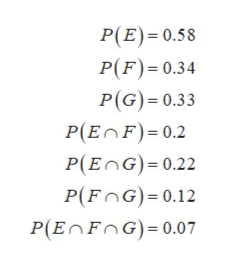# Let E, F and G be three events in S with P(E) = 0.58, P(F) = 0.34, P(G) = 0.33, P(E ∩ F) = 0.2, P(E ∩ G) = 0.22, P(F ∩ G) = 0.12, and P(E ∩ F ∩ G) = 0.07. Find P(EC ∩ F ∩ G), P(E ∩ FC ∩ G), and P(E ∩ F ∩ GC).

Question
50 views

Let EF and G be three events in S with P(E) = 0.58, P(F) = 0.34, P(G) = 0.33, P(E ∩ F) = 0.2, P(E ∩ G) = 0.22, P(F ∩ G) = 0.12, and P(E ∩ F ∩ G) = 0.07. Find P(EC ∩ F ∩ G), P(E ∩ FC ∩ G), and P(E ∩ F ∩ GC).

check_circle

Step 1

Consider E, F and G be three independent events in sample space, S.help_outlineImage TranscriptioncloseP(E) 0.58 P(F) 0.34 P(G) 0.33 P(EnF) 0.2 P(EnG) 0.22 P(FnG) 0.12 P(EnFnG) 0.07 fullscreen
Step 2

The complements of events E, F and G can be obtained as:

Step 3

The required probability c...

### Want to see the full answer?

See Solution

#### Want to see this answer and more?

Solutions are written by subject experts who are available 24/7. Questions are typically answered within 1 hour.*

See Solution
*Response times may vary by subject and question.
Tagged in

### Basic Probability